# Asymptotic expansion

(diff) ← Older revision | Latest revision (diff) | Newer revision → (diff)

of a function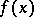A series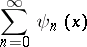such that for any integerone has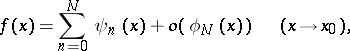(1)

whereis some given asymptotic sequence as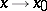. In such a case one also has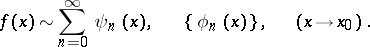(2)

The sequence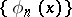is omitted from formula (2) if it is clear from the context which sequence is meant.

The asymptotic expansion (2) is called an asymptotic expansion in the sense of Erdélyi . An expansion of the type(3)

where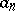are constants, is called an asymptotic expansion in the sense of Poincaré. If the asymptotic sequence of functionsis given, the asymptotic expansion (3), contrary to the expansion (2), is uniquely defined by the functionitself. If (1) is valid for a finite number of values, then (1) is called an asymptotic expansion up to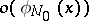. The series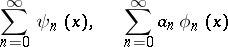are known as asymptotic series. As a rule such series are divergent. Asymptotic power series are the ones most commonly employed; the corresponding asymptotic expansions are asymptotic expansions in the sense of Poincaré.

The following is an example of an asymptotic expansion in the sense of Erdélyi: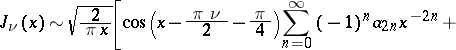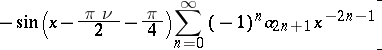, whereis the Bessel function, andThe concepts of an asymptotic expansion of a function and of an asymptotic series were introduced by H. Poincaré  in the context of problems in celestial mechanics. Special cases of asymptotic expansions were discovered and utilized as early as the 18th century . Asymptotic expansions play an important role in many problems in mathematics, mechanics and physics. This is because many problems do not admit exact solutions, but their solutions can be obtained as asymptotic approximations. Moreover, numerical methods are often disregarded if asymptotic approximations can be relatively easily found.

How to Cite This Entry:
Asymptotic expansion. Encyclopedia of Mathematics. URL: http://encyclopediaofmath.org/index.php?title=Asymptotic_expansion&oldid=16660
This article was adapted from an original article by M.V. Fedoryuk (originator), which appeared in Encyclopedia of Mathematics - ISBN 1402006098. See original article